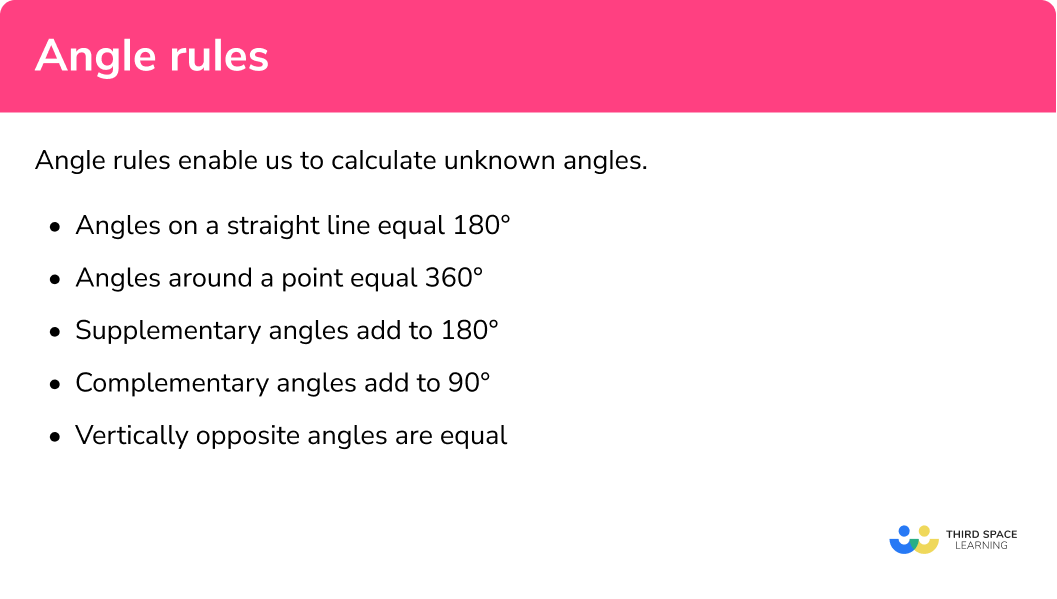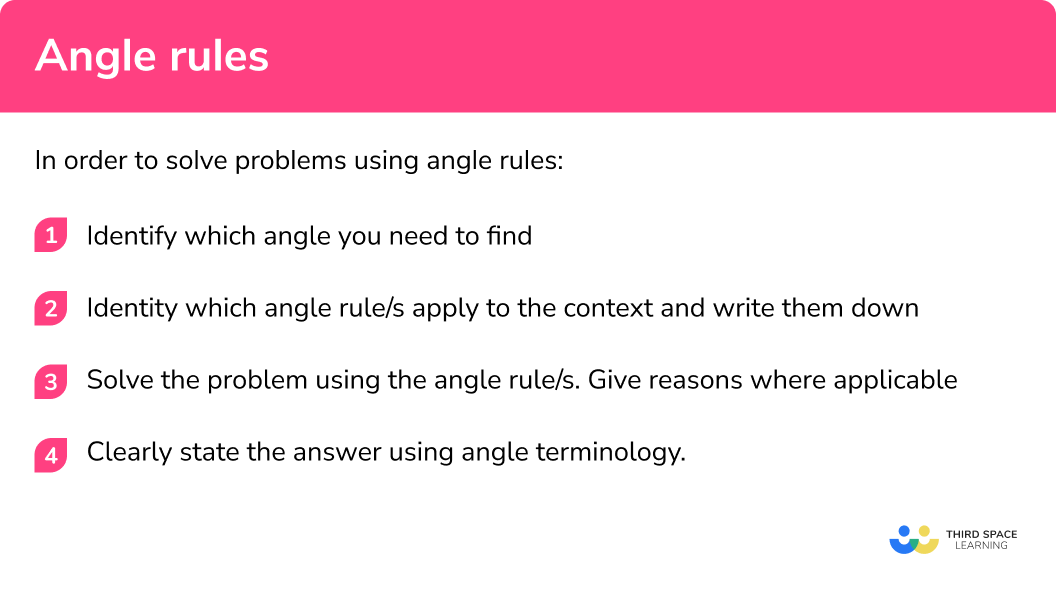# Angle Rules

Here we will learn about angle rules including how to solve problems involving angles on a straight line, angles around a point, vertically opposite angles, complementary angles and supplementary angles.

There are also angle rules worksheets based on Edexcel, AQA and OCR exam questions, along with further guidance on where to go next if you’re still stuck.

## What are angle rules?

Angle rules enable us to calculate unknown angles:

• Angles on a straight line equal 180º
Angles on a straight line always add up to 180º.

E.g.

Here the two angles are labelled 30º and 150º . When added together they equal 180º and therefore lie on a straight lie. These angles share a vertex.

However, below we can see an example of where two angles do not equal 180º:

This is because they do not share a vertex and therefore do not lie on the same line segment.

Note: you can try out the above rule by drawing out the above diagrams and measuring the angles using a protractor.

Step-by-step guide: Angles on a straight line

• Angles around a point equal 360o
Angles around a point will always equal 360º.

E.g.

The three angles above share a vertex and, when added together equal 360o.

Step-by-step guide: Angles around a point

• Supplementary angles
Two angles are supplementary when they add up to 180º , they do not have to be next to each other.

E.g.

These two angles are supplementary because when added together they equal 180º.

Step-by-step guide: Supplementary angles

• Complementary angles
Two angles are complementary when they add up to 90º , they do not have to be next to each other.

E.g.

These two angles are supplementary because when added together they equal 90º.

Step-by-step guide: Complementary angles

• Vertically opposite angles
Vertically opposite angles refer to angles that are opposite one another at a specific vertex and are created by two lines crossing.

E.g.

Here the two angles labelled ‘a’ are equal to one another because they are ‘vertically opposite’ at the same vertex.

The same applies to angles labelled as ‘b’.

Step-by-step guide: Vertically opposite angles

When solving problems involving angles sometimes we use more than the above rules. Below you will see a range of problems involving angles with links to lessons that will go into more detail with more complex questions.

### What are angle rules?### Keywords

It is important we are familiar with some key words, terminology and symbols for this topic:

• Angle: defined as the amount of turn round a common vertex.
• Vertex: the point created by two line segments (plural is vertices).

We normally label angles in two main ways:

1By giving the angle a ‘name’ which is normally a lower case letter such as a, x or y or the greek letter θ (theta). See below for an example:

2By referring to the angle as the three letters that define the angle. The middle letter refers to the vertex at which the angle is e.g. see below for the angle we call ABC:

## How to use angle rules

In order to solve problems involving angles you should follow these steps:

1. Identify which angle you need to find.
2. Identity which angle rule/s apply to the context and write them down.
3. Solve the problem using the above angle rule/s. Give reasons where applicable.
4. Clearly state the answer using angle terminology.

### How to use angle rules## Angle rules examples

### Example 1: angles on a straight line

Find angles a and b.

1. Identify which angle you need to find (label it if you need to).

You need to find angles labelled a and b.

2Identify which angle rule/s apply to the context and write them down (remember multiple rules may be needed).

Angles on a straight line at the same vertex always add up to 180o.

Notice how angles a and b do not share a vertex.

3Solve the problem using the above angle rule/s. Give reasons where applicable.

\begin{aligned} a+110&=180 \\ a=&70 \end{aligned}

\begin{aligned} b+55&=180 \\ b&=125 \end{aligned}

4Clearly state the answer using angle terminology.

Angle a = 70°

Angle b = 125°

### Example 2: angles around a point

Find the size of θ:

You need to find the angle labelled θ (theta).

All the angles are around a single vertex and we know that angles around a point equal 360o.

\begin{aligned} \theta+120+95+30&=360 \\ \theta+245&=360 \\ \theta&=115 \end{aligned}

$\text{Angle } \theta = 115^{\circ}$

### Example 3: using supplementary angles

Two angles are supplementary and one of them is 127°. What is the size of the other angle?

You need to find the other angle in a pair of supplementary angles where one is 127°. We will call this angle ‘a’.

Supplementary angles add up to 180°.

\begin{aligned} 127 +a &= 180\\ a&=53\\ \end{aligned}

The other angle is 53°.

### Example 4: using complementary angles

If two angles are complementary and one of them is 34°, what is the size of the other angle?

You need to find the other angle in a pair of supplementary angles where one is 34°. We will call this angle ‘b’.

Complementary angles add up to 90°.

\begin{aligned} 34 + b &=90\\ b &= 56\\ \end{aligned}

The other angle is 56°.

### Example 5: vertically opposite angles

Find angle BCD.

Find the angle at vertex C made from lines CD and CB. The diagram shows it labelled as x

Angle x and 80are vertically opposite one another at a vertex which has been created by two lines crossing.

Vertically opposite angles are equal to one another

x = 80 because they are vertically opposite

Angle BCD = 80°

### Example 6: Applying multiple rules to solve a problem

In the diagram below:

• Angle AOB is a right angle.
• AOE and EOD are complementary angles.
• Angle AOE is 50 degrees.

Find angle COD.

Let’s start by labelling the diagram. We have labelled the angle we are trying to find x.

Two angles are complementary when they add up to 90o.

Angles around a point will always equal 360o.

Angles on one part of a straight line always add up to 180o.

Vertically opposite angles are equal.

Note: there are multiple ways we can solve this problem. Below is just one method.

As AOE and EOD are complementary angles then they must equal 90 degrees. Therefore:

\begin{aligned} 50+E O D&=90 \\ E O D&=40 \end{aligned}

EOD and BOC are vertically opposite. Therefore:

\begin{aligned} B O C&=E O D \\ B O C&=40 \end{aligned}

Angles around a point are equal to 360 degrees. Therefore:

\begin{aligned} A O B+A O E+E O D+B O C+x=360 \\ 90+50+40+40+x=360 \\ 220+x=360 \\ x=140 \end{aligned}

Angle COD = 140°

### Common misconceptions

•  Incorrectly labelling angles
•  Misuse of the ‘straight line’ rule where angles do not share a vertex
•  Mixing up the rules for supplementary and complementary angles
•  Finding the incorrect angle due to misunderstanding the terminology

### Practice angle rules questions

1. Find angle xx=30^{\circ}x=60^{\circ}x=90^{\circ}x=180^{\circ}Using angle on a straight line we have 180 – (90 + 30) = 60^{\circ}

2. Can angles 40^{\circ}, 100^{\circ}, 115^{\circ}, 105^{\circ} lie around a single point?

YesNoThe sum of these angles is 360 .

3. Find angle a:a=30^{\circ}a=60^{\circ}a=90^{\circ}a=50^{\circ}Using angles around a point, we have 360 – (125 + 125 + 50) = 360 – 300 = 60^{\circ}

4. Are angles 60^{\circ} \text{ and } 90^{\circ} supplementary angles?

YesNoThe sum of these angles is not 180 .

5. Are angles 75^{\circ} \text{ and } 15^{\circ} complementary angles?

YesNoThe sum of these angles is 90 .

6. Find angle zz=115^{\circ}z=60^{\circ}z=65^{\circ}z=55^{\circ}Vertically opposite angles are equal

### Angle rules GCSE questions

1. Work out the size of angle z .(2 marks)

360-169-83

(1)

108^{\circ}

(1)

2.(a) Find the size of angle a .
(b) Find the size of angle b .

(3 marks)

a)

49^{\circ}

(1)

b)

180-49

(1)

131^{\circ}

(1)

3. Work out the size of angle x . Give reasons for your answer.(3 marks)

180-90-57=33

(1)

Angles on a straight line add up to 180^{\circ}.

(1)

x=33^{\circ}

(1)

## Learning checklist

You have now learned how to:

•  Use conventional terms and notation for angles
• Apply the properties of angles on a straight line, around a point and on vertically opposite angles
• Apply angle facts and properties (e.g. supplementary and complementary angles) to solve problems

## Still stuck?

Prepare your KS4 students for maths GCSEs success with Third Space Learning. Weekly online one to one GCSE maths revision lessons delivered by expert maths tutors.

Find out more about our GCSE maths tuition programme.